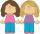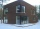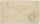# Bus vs. train

Bus started from point A 10 minutes before the train started from the same place. The bus went an average speed of 49 km/h, train 77 km/h.
To point B train and bus arrived simultaneously.

Calculate time of train journey, if train and bus travelled the same distance.

Result

t =  17.5 min

#### Solution:49 (t+10) = 77 t

28t = 490

t = 352 = 17.5

Calculated by our simple equation calculator.

Leave us a comment of example and its solution (i.e. if it is still somewhat unclear...):

Showing 0 comments:Be the first to comment!#### To solve this verbal math problem are needed these knowledge from mathematics:

Do you have a linear equation or system of equations and looking for its solution? Or do you have quadratic equation? Do you want to convert length units?

## Next similar examples:

1. Train delayThe train should be in the station for 10 minutes. It still has 32 km left. What is the expected delay if the train travels in 2 minutes 3 km except for the last section where it is 2 km in 5 minutes.
2. SistersSisters Hanka and Vera goes mostly tograndmother by bicycle. Journey took Hanka 30 minutes and Vera 20 minutes. How long will Vera catch up with Hanka when started from home five minutes later than Hanka?
3. InfirmaryTwo thirds of children from the infirmary went on a trip seventh went to bathe and 40 children remained in the gym. How many children were treated in the infirmary?
4. Bus 14Boatesville is 65.35 kilometers from Stanton. A bus traveling from Stanton is 24.13 kilometers from Boatesville. How far has the bus traveled?
5. Dropped sheetsThree consecutive sheets dropped from the book. The sum of the numbers on the pages of the dropped sheets is 273. What number has the last page of the dropped sheets?
6. Bob traveledBob traveled 20 meters to Dave's house. He arrived in 3 minutes. What was his average speed for the trip.
7. One-thirdA one-third of unknown number is equal to five times as great as the difference of the same unknown number and number 28. Determine the unknown number.
8. Equation 29Solve next equation: 2 ( 2x + 3 ) = 8 ( 1 - x) -5 ( x -2 )
9. How oldThe student who asked how many years he answered: "After 10 years I will be twice as old than as I was four years ago. How old is student?
10. Family 8Father is 38 years old, daughter 12, son 14. How many years will father have as many years as his children together?
11. SymbolsIf 2*3 = 60 ; 3*4 = 120 and 4*5 = 200, what is 2*5?
12. TimeageSeven times of my age is 8 less than the largest two-digit number. How old I am?
13. Unknown numberIdentify unknown number which 1/5 is 40 greater than one tenth of that number.
14. Cyclist 9A cyclist travels at a a speed of 4.25 km per hour. At that rate, how far can he travel in 3.75 hours?
15. Find xSolve: if 2(x-1)=14, then x= (solve an equation with one unknown)
16. Six yearsIn six years Jan will be twice as old as he was six years ago. How old is he?
17. AuntAnnie aunt is 35 years old, Annie is 14 years. For how many years will aunt have twice as many years as Annie?